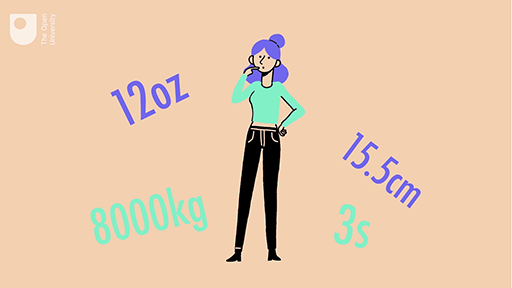Science, Maths & Technology

### Become an OU studentEveryday maths 1 (Wales)

Start this free course now. Just create an account and sign in. Enrol and complete the course for a free statement of participation or digital badge if available.

# Session 2: Units of measure

## Introduction

You come across problems requiring calculation every day. These problems could be related to money, time, length, weight, capacity and temperature. For example, if you were buying a new washing machine, you would measure the space where you want to put it under your worktop and make sure you chose a washing machine short enough to fit the space.

In this session of the course you will find out about measuring and calculating length, distance, weight, capacity (volume), temperature and time. You will learn how to use different metric measurements, such as kilometres, metres and centimetres, grams and kilograms, and litres.

By the end of this session you will be able to:

• measure and understand the sizes of objects
• read a mileage chart to find the distance between places
• find out how heavy things are and understand weights
• measure and understand volumes and capacities
• measure and compare temperatures
• express time using the 24-hour clock
• carry out calculations with time.